Mathematical and Physical Journal
for High Schools
Issued by the MATFUND Foundation
 Already signed up? New to KöMaL?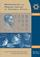# English Issue, December 2002

Previous pageContentsNext pageORDER FORM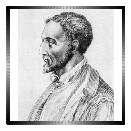## Cardan and cryptographyThe mathematics of encryption grids

To the 500th anniversary of the Girolamo Cardano

Tamás Dénes

### Introduction

The 16th century had just begun when Girolamo Cardano (1501-1576), Italian mathematician, physicist, philosopher, physician (a real renaissance scholar) was born. His 1545 publication titled Ars Magna contains general formulae for the roots of the cubic equation. Today, it is these formulae that Cardan's name is most often associated with, though it is still uncertain whether these discoveries were his own.1

Very few of us recognize Cardan as one of the most outstanding figures of 16th-century cryptography. This is not so surprising, as it is natural that cryptography should be pursued inconspicuously. The strictly confidential correspondence of kings and warlords used various cipher systems. As the more complicated cipher techniques, and especially the decryption of messages often require advanced mathematical skills, it can be expected that the theoretical background should be established in a large part by famous mathematicians.

Cardan developed a cipher system, then completely unknown, that is now called Cardan's screen. The success of Cardan's encryption screen is best proved by the fact that is was still used 400 years later, in the middle of the 20th century, by the West-German intelligence service (BND = Federal Information Service.)

In this paper, after a short historical overview, we illustrate the principle of Cardan's encryption screen, and then discuss several generalizations and a few mathematical properties of the grid.

### Torch telegraphy and interval cipher

Cardan conducted a thorough research of the cipher systems of the past, back to antiquity. He found a text by Polybius, a Greek historian of the 2nd century BC, in which the author describes an interesting and completely unusual technique.

### Torch telegraphy by Polybius

Consider the 5 by 5 table in Figure 1.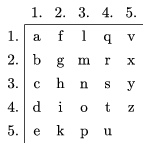Figure 1

The sender of the message needs 10 torches, 5 for each hand. He sends the message letter by letter, by holding up as many torches with his left hand as the number of the row, and with his right hand as the number of the column containing the letter to be sent. For example, in the case of the letter s'', he holds 3 torches in his left hand and 4 in his right hand. Polybius was very proud of his method:

This method was invented by Cleoxenus and Democritus but it was enhanced by me'', he wrote.

He was proud with good reason. Though the idea of sending messages by means of torches was known and used by the ancient Chinese2 a lot before Polybius, his cipher was the first to apply a table. The great advantage of a table is that the alphabet or the arrangement of the letters in the table can be changed any time without changing the method itself.

Question: In how many different ways can the 24 letters of the alphabet be arranged in Figure 1? What if all the 25 fields are filled up?

Cardan further improved this method by reducing the number of torches to two, one in each hand. The letters of the alphabet were coded by the respective positions of the torches. This method may have given Cardan the idea of two new types of cipher systems.

One type is interval cipher'' in which the message is coded by distances between letters. For simplicity, let us illustrate the method by an example. Prepare a table identical to that Figure 2.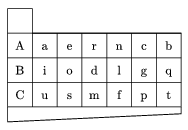Figure 2

Let the message be piglet''. (The steps of the procedure can be followed in Figures 3(a) and 3(b).) Take a blank sheet of paper. In the upper left corners, write any one of letters A, B, C (we choose C). This will only mark the beginning of the text. Take the table of Figure 2, and place the blank field onto the beginning letter.

Locate the first letter of piglet'' (p) in the table, and write the symbol of the row (C) directly above the letter p (Figure 3(a)).

[htb]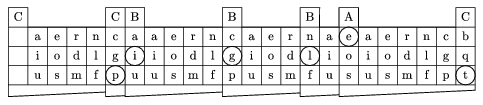Figure 3(a)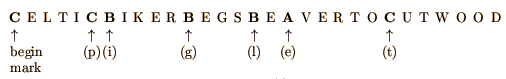Figure 3(b)

Now place the blank square of the table onto the last capital letter written over the table, and locate the next letter (i) of the message. Repeat the previous procedure: Write the symbol (B) of the row over the letter i of the table. The procedure is continued until there is no more room left in the current row of the sheet. Then the first step is repeated and another row is opened. And so on, until the message is over. We can make the task of decryption even harder by filling up the spaces with arbitrary letters different from A, B, C (Figure 3(b).) (It is even better to complete the cipher to a meaningful text, but that is not necessary.)

The receiver of the message holds an identical table to that used by the sender. Thus by carrying out the above procedure starting at the begin mark, the receiver is able to read the message.

The modern reader might find this an interesting technique but it seems impractical, as the use of the table is complicated, and it requires too much space for its relatively small amount of information content. Cardan's method was also criticized by his contemporaries for being hard to follow. They did not realize that Cardan was far ahead of his age by establishing what is now called a non-symmetrical cipher. As opposed to other methods in use at that time, the above procedure is more than a simple substitution cipher.3 It is a one-to-many rather than a one-to-one assignment, as the three capital letters code 6 lowercase letters each. Extra information is needed to decrypt the message (the position of the letter in the line, i.e. its distance from the reference letter). Consequently, unlike in simple substitution, the frequencies of the letters in the ciphertext do not match the frequencies in the plain text.

### Cardan's screen

The other type of cipher system invented by Cardan, which bears his name to this day, uses the so-called Cardan grid (screen). The encryption grid is a matrix of letters. For illustration, let us cite Cardan's own words.4

Take two sheets of parchment of the same size, ruled for writing, and on the lines of both make slits at various places. These slits are to be small, but of proper size for the size or height of letters of the alphabet. Some of the slits will hold seven, some three, some eight or ten letters, so that all the slits together will hold 120 letters, counting all the letters which can be inserted in them. One of these sheets of parchment you will give to your correspondent. When occasion arises, first write your message as briefly as possible, in such a way that the message may consist of a smaller number of letters than the slits will hold. Then write your message on a sheet of parchment placed beneath the slits, and again on a second sheet, and on still a third. Then fill the spaces of the first sheet by completing sentences, erasing, and filling in until connected sense results. Next, on the second sheet, finish the message which you now have in such a way that the words and sentences may appear coherent. Arrange it again on the third sheet of parchment in such a way that, without disturbing the original letters, the entire sense and the number and size of the words may hang together and retain a harmony of style. When this is completed, lay the sheet containing the slits upon a sheet of the same size, and place minute dots at the ends of the slits to mark the limits of the letters which you wish to insert. Then take the third sheet of parchment [i.e., the final draft of the three which have been made] and copy the message from it with the words in regular order and with a proper arrangement of spaces and size of the letters, so that the original [i.e., the secret] message and its words may be contained within the limits marked by the dots. No suspicion of any deception will now remain. Your correspondent, when he receives your communication, places his slitted sheet of parchment over it and reads what you wish to convey. Although this method entails no slight labor, none equally good can be devised for conveying information to friends in dangerous times.''

The encryption screen became remarkably popular in cryptography. The success of the technique may have been due to its simplicity and versatility together. Such a success occurs very rarely in the history of science, especially in cryptography. With the development of technical skills, cryptanalysis usually catches up with cryptography. Though Cardan's name did not become widely known in this area, his cipher screen remained in use for 450 years. It has even found its way to fiction: In Jules Verne's Mathias Sandorf, the villains Torontal and Sarcany intercept Sandorf's coded message and decipher it by getting hold of a copy of the screen. The screen appears right at the beginning of the novel, and becomes an important factor of the plot. The example below illustrates a 6x6 grid. The table of Figure 4 has been filled in with the sentence (O) how sweet to be a cloud floating in the blue.'' It is interesting to see how many different messages may be hidden in this ciphertext. The bold numbers in Figures 5, 6, 7 stand for the windows in Cardan's screen. If the screen is placed over the table of Figure 4, the letters shown in the windows reveal the encrypted message (when read left to right, row by row.)

The ciphertext in Figure 4: O HOW SWEET TO BE A CLOUD FLOATING IN THE BLUE.

The message revealed by the screen of

Figure 6: HOT COD FAT HUE.

Figure 7: WEE TOE OF ANNE.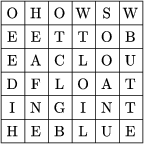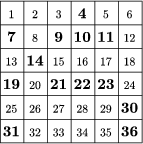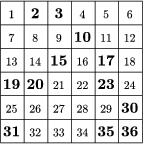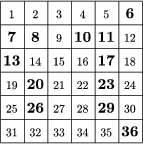Figure 4 Figure 5 Figure 6 Figure 7

The text written in an nxn table (n rows and n columns) can be n2 letters long. If the length of the message is k letters (where obviously k<n2) then the number of grids containing k windows is

$\displaystyle {n^2\choose k}=\frac{n^2!}{k!(n^2-k)!}.$

To see how large this number is, consider the grid of Figure 6. Here n=6, k=12, the number of possible grids is therefore

$\displaystyle \frac{36!}{12!\cdot24!}=31\cdot29\cdot28\cdot25\cdot17 \cdot13\cdot9=1\;251\;677\;700.$

The number of grids can be calculated in a similar way for Figure 5 (where n=6, k=13).

### Let us rotate the grid

In the simple Cardan screen described above, the choice of the positions of the windows was arbitrary. Let us consider now another technique for preparing the screen and filling out the table: the rotating screen. The rotating screen is an encryption device that can be rotated through 90 degrees about the center of the corresponding letter matrix, revealing each field in exactly one of the four positions.

The choice of the window positions is not arbitrary any more, as no field can be revealed by more than one window during the rotations. Each window has to have a different position after every rotations. It is clear that, for a rotating screen, n, the size of the matrix has to be even, as $\displaystyle k=\frac{n^2}{4}$ is the number of windows needed for revealing each field in exactly one of the four positions of the screen.

Let us illustrate the rotating screen with an example. Let n=6 be the size of the grid. Thus we need 9 windows.

Step 1: Divide the 6x6 matrix into four zones as shown in Figure 8.

Step 2: Select k1 fields from zone I, where 1$\displaystyle \le$k1$\displaystyle \le$9. We have k1=2, and fields 4 and 6 are selected. (See Figure 9.)

Step 3: Rotate the grid through 90 degrees and mark those fields of zone II that the chosen fields (4 and 6) cover. Then go on rotating the grid through 90 and mark the covered fields in each position. (See Figure 9.) Thus the choice of k1 windows makes 4 k1 fields covered.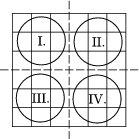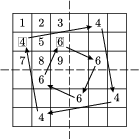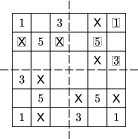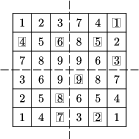Figure 8 Figure 9 Figure 10 Figure 11

Step 4: Now select k2=3 fields, still uncovered, from zone II. We have selected fields 1, 3 and 5. (See Figure 10 where X marks the covered fields, the boxes around the numbers mark the windows, and the numbering of the fields of the zone corresponds to the rotated positions of the fields of zone I.) With the four rotations 4 k2 more fields are covered.

Step 5: Repeat the above procedure in zones III and IV, too. Then 4k1+4k2+4k3+4k4=n2, and we get k=k1+k2+k3+k4=9 for the number of windows.5 (See Figure 11 where the boxed numbers represent the windows.)

Note that we are free to choose each of the k windows from any of the zones I-IV, and the number of all possible rotating nxn screens (with the maximum number of windows) is therefore $\displaystyle 4^k=4^{\frac{n^2}{4}}$. In our example, that is 49=262 144.

Question: For a given grid size n and window number k, are there more simple screens or more rotating screens?

For the same grid size, there are much more simple screens than rotating screens. This comparison however is misleading from the cryptographical point of view, as the rotating screen makes it possible to write the letters of the plain text in the whole matrix6, whereas the simple screen only uses a part of it.

As an illustration, see the Figures 12, 13, 14, 15 for the encryption of the plain text in Figure 3 using the screen of Figure 11.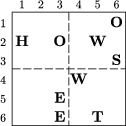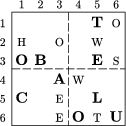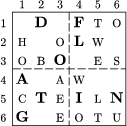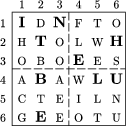Figure 12 Figure 13 Figure 14 Figure 15

Step 1: On a blank sheet, draw a grid of the same size as the screen, and place the screen on the grid.

Step 2: Fill in the windows with the first nine letters of the plain text, proceeding left to right, row by row.

Step 3: Rotate the screen through 90 degrees, and repeat Step 2.

Step 4: Repeat Step 3 two more times.

The result is the letter matrix of Figure 15 that is sent to the recipient, who can decrypt the message with an identical screen following Steps 1-4.

(Another interesting feature of our example is that with the simple screens of Figures 5, 6, 7, further hidden messages can be revealed from the decrypted text.)

It is an interesting historical addition to Cardan's screen that exactly 250 years after Cardan's Ars Magna, the German mathematician Carl Friedrich Hindenburg published a book7 in which the entire Chapter VI focuses on cryptography, and on encryption screens in particular. He does not even mention Cardan's name (which is not unusual in the history of science and technology). Besides providing a detailed description of Cardan's screen, Hindenburg's book also shows possible improvements. It points out that if the sides of the screen are labelled by the letters a, b, c, d then the rotations of the screen can be represented by permutations of the four letters. This increases the number of possibilities by a factor of 4!=24.

Question: How does the permutation change the encryption algorithm?

As we shall see below, apart from their use in the process of preparing the grid permutations also play a role in the selection of the windows of the screen.

### Permutations, Latin squares and encryption screens

A Latin square is an nxn square matrix whose rows and columns are permutations of the numbers 1,2,...,n.

An nxn matrix is a permutation matrix if it contains exactly n 1's such that there is exactly one 1 in each row and column, and the remaining entries are all zeros. The following simple result is important from the point of view of encryption grids:

Every nxn Latin square L(n) can be represented in one unique way in terms of n permutation matrices as follows:

 (1) L(n)=1.P1+2.P2+...+n.Pn,

Moreover, the 1's in the permutation matrix Pk appear in the positions where the Latin square L(n) contains the number k.

(The operation of matrix addition as well as the multiplication of a matrix by a number is performed entry by entry.)

The permutation matrices obtained in this way can be used as encryption screens. The technique is illustrated by the following example:

$\displaystyle L(4)=\left[\matrix{1&2&3&4\cr3&4&2&1\cr2&1&4&3\cr4&3&1&2} \right]$

$\displaystyle P_1=\left[\matrix{1&0&0&0\cr0&0&0&1\cr0&1&0&0\cr0&0&1&0} \right],\quad P_2=\left[\matrix{ 0&1&0&0\cr0&0&1&0\cr1&0&0&0\cr0&0&0&1}\right],\quad P_3= \left[\matrix{ 0&0&1&0\cr1&0&0&0\cr0&0&0&1\cr0&1&0&0}\right],\quad P_4= \left[\matrix{ 0&0&0&1\cr0&1&0&0\cr0&0&1&0\cr1&0&0&0}\right].$

Each permutation matrix Pi represents an encryption screen whose windows are in the positions of the 1's. Now, instead of rotation, one should place the permutation matrices as encryption screens over the letter matrix in order to encrypt or decrypt the message. As the theorem above ensures that the resolution into permutation matrices is unique, the windows of the screens thus produced reveal each of the n2 fields exactly once, and therefore the entire letter matrix can be filled with the plain text, just like with rotating screens. As a further advantage, the uniqueness of the resolution of the Latin square remains valid if the order of the permutation matrices (screens) is changed. Thus the number of possibilities is n! times the number of resolutions.8

The problem with the practical application of encryption screens is that they require a lot of space, as the entire permutation matrix is needed for writing n characters into the letter matrix. This, being a binary matrix, takes n2 bytes to be stored. If the permutation matrices are numbered, i.e. every nxn permutation matrix is assigned to a permutation of the numbers 1,2,...,n then the required memory space is reduced.

This kind of numbering only requires the positions of the 1's, as all the other entries are zeros. Suppose the entry in the i-th row and j-th column is 1. Then set the i-th element of the corresponding permutation equal to j. Since there is a single 1 entry in each row of the matrix the result is a permutation indeed.

We can apply an appropriate algorithm to assign numbers 1 to n! to the permutations. If the permutation matrix is used as an encryption screen, then, instead of the actual matrix and the corresponding permutation it is enough to send its number along with the ciphertext.

Storing a permutation of the numbers 1,2,...,n requires c(n) characters where9

 (2) c(n) = ( [log n] + 1)n

The number of digits of the number n! (denoted by j(n)) is

 (3) j(n)=[log n!+1].

Hence

 (4) $\displaystyle \frac{j(n)}{c(n)}=\frac{[\log n!+1]}{n\big([\log n]+1\big)}=\frac{\sum\limits_{i=1}^n{\log i}+1}{n\big([\log n]+1\big)},$

and thus

 (5) $\displaystyle \lim_{n\to\infty}\frac{j(n)}{c(n)}=1.$

However, for typical values of n, the above assignment is still useful in practice. The table below shows the values of j(n) and c(n) and that we can save 25-50% of memory space by storing and sending the number of the permutation instead of the permutation itself.

 n j(n) c(n) j(n)/c(n) 10 7 20 .35 100 158 300 .526 200 375 600 .625 300 615 900 .683 400 869 1200 .724 500 1134 1500 .756

Table 1

### A generalization of Cardan's screen: the k-screen (cobweb screen)

Both Cardan and Hindenburg used square screens. Now we show that the technique can be extended to arbitrary regular n-sided polygons.

Consider the construction in Figure 16. It is a regular polygon of k sides. Each side is $\displaystyle \frac{N}{2}$ units long, that is, the outermost layer of each sector consists of $\displaystyle N-1\left({ =\frac{N}{2}+\frac{N}{2}-1}\right)$ cells. There are $\displaystyle \frac N2$ layers in a sector, and N-(2i-1) cells in the i-th layer. Hence the total number of cells in each layer is $\displaystyle \sum\limits_{i=1}^{\frac{N}{2}}N-(2i-1)=\frac{N^2}{4}$. Thus the number of cells in the entire k-grid is $\displaystyle k\frac{N^2}4$. (In the case of Cardan's grid, k=4, and the result is the same as the number of cells in the square grid.)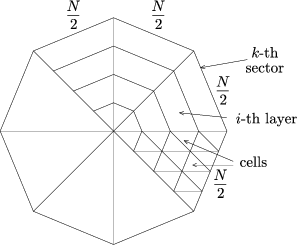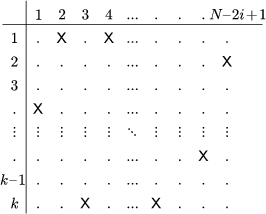Figure 16 Figure 17

Obviously, the angle of rotation of the k-grid is not 90o any more, but $\displaystyle \frac{360^\circ}k$. The real problem is how to choose the positions of the windows in the screen. Consider the matrix below (Figure 17) that has k rows (corresponding to the sectors of the k-grid) and N-2i+1 columns (the number of cells in the i-th layer). The X marks in Figure 17 represent the windows. According to the rules of the rotating screen, there must be exactly one window in each column of the matrix. Hence the number of window combinations in the i-th layer is kN-2i+1.

As the positions of windows in different layers are independent of each other, the total number of window combinations in the k-screen is

 (6) $\displaystyle SC_k^N=\prod_{i=1}^{\frac N2}k^{N-2i+1}=k^{\sum\limits_{i=1}^{\frac N2}N-2i+1}=k^{\frac{N^2}4}.$

It can be seen that for k=4 this equals the number of possible Cardan screens. Hindenburg's permutations can also be applied here, which increases the number of possibilities by a factor of k!. The table below illustrates the number of possible k-screens for a few grid sizes.

 k N SCkN k N SCkN 3 4 81 4 10 1 125 899 906 842 624 3 6 19 683 5 4 625 3 8 43 046 720 5 6 1 953 125 3 10 847 288 598 528 5 8 152 587 894 784 4 4 256 6 4 1 296 4 6 262 144 6 6 10 077 696 4 8 4 294 967 296 6 8 2 821 109 841 920

Table 2

Finally, Figure 18 shows a complete ciphertext matrix and Figure 19 provides the corresponding Cardan screen (where the squares represent the windows). The decryption is left to the reader as an exercise, and the reader can use the screen for producing his own encrypted messages.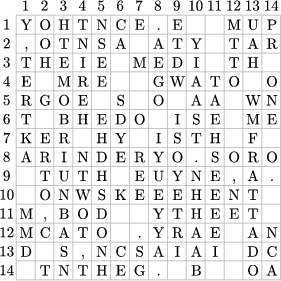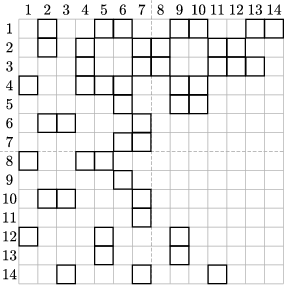Figure 18 Figure 19

1 According to historians, Scipione del Ferro (1465-1526) had found the general solution for cubic equations and showed it to his colleagues. That probably happened around 1515, when mathematical competitions were fashionable in Italy. A colleague of Ferro suggested to Niccolo Tartaglia (1500-1557), a mathematician of great learning, that they should solve cubic equations. Tartaglia solved the equations by the set deadline, but he did not reveal his technique. Cardan asked him so persistently for the method that finally, he confided the solution to Cardan, but he made Cardan swear to secrecy. Cardan broke his word and published the method in his Ars Magna, in 1545. A bitter dispute started between Tartaglia and Cardan, and it remains unsettled to this day.

2 As early as 2000 years ago, the Chinese were able to transmit messages very quickly (and accurately) along the Great Wall with torches held up by men positioned at 100-m intervals.

3 In cryptography, substitution cipher'' means that by some rule, there is one particular (different) letter of an alphabet assigned to the letters of the message. The distribution of letters in the ciphertext will thus be the same as in the plain text.

4 Quoted from Cardan on cryptography, , by Charles J. Mendelssohn.

5 The number of windows can of course be less than that, but then the screen will not reveal all the fields during the rotation, and we cannot use the whole matrix for encryption.

6 Encryption with a simple screen does not change the order of the letters of the plain text, while the rotating screen mixes the letters. Cryptography uses two kinds of encryption: substitution and transposition, and a cipher may use one of the two methods or a combination of them. The great cryptographical advantage of the rotating screen is the combination of the two methods. It should be noted, therefore, that quantity is not the only factor to consider when comparing ciphers.

7 Carl Friedrich Hindenburg: Urchid der Reinenen und Angewandten Mathematic herausgegeben von Carl Friedrich Hindenburg, Leipzig, 1795.

8 For lack of space, we can but briefly mention that every Latin square represents an operation table, which operations, under certain conditions, possess favorable properties for encryption (non-commutative, non-associative). In a future paper we are going to discuss these properties and compare them to the number theoretical cipher systems that are so fashionable today.

9where [x] denotes the greatest integer less than or equal to x.